# Amounts of Substances

## Relative Formula Mass

Calculating relative formula mass

The relative formula mass is JUST the relative atomic mass of all of the atoms in the molecule added up.

Example 1: Magnesium Chloride

MgCl2 has a relative formula mass of 95 since there is one Magnesium (atomic mass 24) and two chloines (each with atomic mass of 35.5).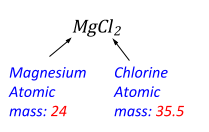Relative Formula Mass of MgCl2 = 24 + 35.5 + 35.5 = 95

Example 2: Dihydrogen Monoxide (Water)

H2O has a relative formula mass of 18 since there is one Oxygen (atomic mass 16) and two Hydrogen atoms (each with atomic mass of 1).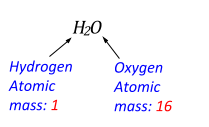Relative Formula Mass of H2O = 1 + 1 + 16 = 18

## Percentage Mass

Calculating percentage mass

This is basically just calculating what percentage of the whole molecule is made out of one particular element.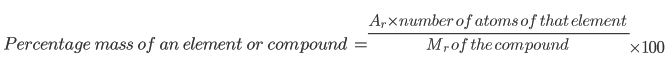Example 1

Find the percentage mass of Chlorine (Cl) in Magnesium Chloride (MgCl2):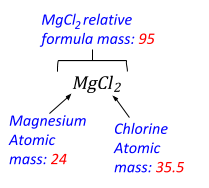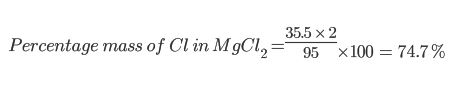Example 2

Find the percentage mass of Chlorine (Cl) in Magnesium Oxide (MgO):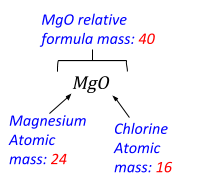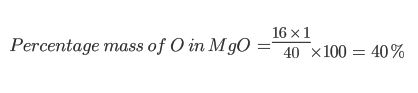Example 3 (HIGHER)

A mixture contains 30% Oxygen by mass. Given that the only compound in the mixture that contains Oxygen is water (H2O), calculate the mass of Water in 100g of the mixture.

Relative atomic masses (Ar): O = 16, H = 1

Step 1: Find the mass of Oxygen in the mixture. Oxygen makes up 30% of the 100g mixture, so find 30% of 100g.

Step 2: Work out the relative formula mass of Water and use this to find the percentage mass of Oxygen in Water:

Mr of water = 16 + 1 + 1 = 18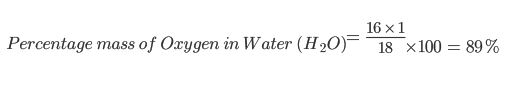Step 3: Calculate what mass of Water contains 30g of Oxygen. Since 30g makes up 89% of the whole mass of the water molecules, then you just need to find what 100% is. Do this by finding 1% and multiplying by 100.

So 1% of the Water mass = 30g / 89 = 0.337g

So 100% = 0.337 x 100 = 33.7g

## Avagadros Constant

Avagadros number is literally just a number (but it’s not any old number). It’s the amount of particles are in 1 mole of substance.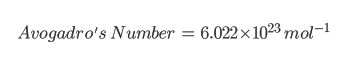The 10 23 just means that it is multiplied by 10, twenty three times. So the number is actually 602200000000000000000000 (but this is very long so we write it in the form above)!

## The Mole

In chemistry, we measure the amounts of substances in Moles. We shorten ‘mole’ to ‘mol’ when it is a unit.

The mass of 1 mole of a substance (measured in grams, g) is exactly the same number as the molecular mass.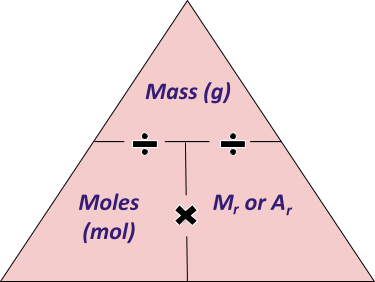For example:

1 mol of Carbon atoms has a mass of 12g (Carbon has an atomic mass of 12)

There are 18g in 1 mol of Water H2O (Oxygen has an atomic mass of 16 and each hydrogen has an atomic mass of 1. 16 + 1 + 1 = 18)

1 mol of Carbon Dioxide CO2 has a mass of 44g (since is one Carbon and two Oxygens, having atomic masses 12 + 16 + 16 = 44)

2 mol of Carbon Dioxide has a mass of 88g (since 1 mole has a mass of 44g and there are 2 moles: 44g + 44g = 88g)

It is useful to remember and learn how to use this equation:

Mass (in grams) = Number of Moles x Mr of compound

Which could be written as a triangle, like so:Examples: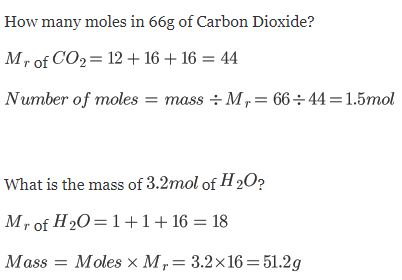## Conservation of Mass

The law of conservation of mass:

During a chemical reaction, particles cannot be created or destroyed. The mass of the products equals the mass of the reactants.

A chemical reaction is basically just a reordering of atoms and bonds! The atoms basically just get shuffled around! No atoms are created or destroyed, so the weight of the substance at the start of the reaction will always be the same as at the end of the reaction. Since the atoms are not being created or destroyed, the total Mr is the same before and after the reaction.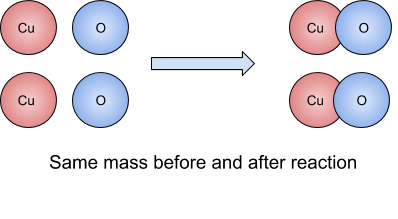Examples:

1. 32g of Copper reacts with Oxygen to form 42g of Copper Oxide. What is the mass of Oxygen that reacts?

42g - 32g = 10g of Oxygen

Sometimes it mas SEEM like the mass is changing:

If it looks like mass is decreasing: This may be because one of the products is a gas, which then drifts away. No matter what though, if you had a completely sealed container, you would see that the mass does not change. You could do this by placing the reacting substances on a weighing scale!

If it looks like mass is increasing: This may be because one of the reactants is a gas. We cannot properly weigh gas on a weighing scale since it’s drifting around in the air. However, the mass is 100% not changing!